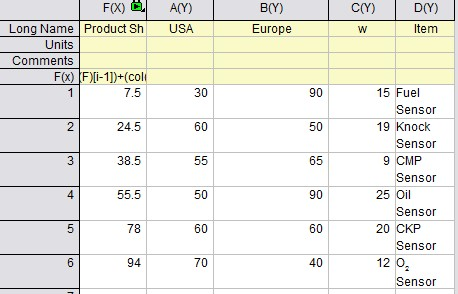# Variable Column/Bar Width

Product Page of Column and Bar Plot

## Variable Column/Bar Width (ORG-4155)

After plotting Stacked Column Graph, e.g from the following data. Here, %Share in column F is not the actual data, but the calculated X center positions of columns using the Set Column Formula:

i==1?(col(C)[i]/2):(col(F)[i-1])+(col(C)[i-1]/2)+(col(C)[i]/2)

The actual data is presented as the column width stored in the column C("w").To set the column width from a dataset, set the dataset to Width field in Spacing tab in Plot Details. You also need to set the Scaling Factor to 0, which is the new 9.1 feature (ORG-6010) to indicate the width data  or symbol size data from worksheet is in the same unit as the X axis.The graph will turn into the following: